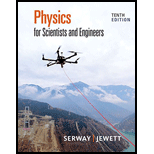# For the circuit shown in Figure P27.22, we wish to find the currents I 1 , I 2 , and I 3 . Use Kirchhoff’s rules to obtain equations for (a) the upper loop, (b) the lower loop, and (c) the junction on the left side. In each case, suppress units for clarity and simplify, combining the terms. (d) Solve the junction equation for I 3 . (e) Using the equation found in part (d), eliminate I 3 from the equation found in part (b). (f) Solve the equations found in parts (a) and (e) simultaneously for the two unknowns I 1 and I 2 . (g) Substitute the answers found in part (f) into the junction equation found in part (d), solving for I 3 . (h) What is the significance of the negative answer for I 2 ? Figure P27.22### Physics for Scientists and Enginee...

10th Edition
Raymond A. Serway + 1 other
Publisher: Cengage Learning
ISBN: 9781337553278

#### Solutions

Chapter
Section### Physics for Scientists and Enginee...

10th Edition
Raymond A. Serway + 1 other
Publisher: Cengage Learning
ISBN: 9781337553278
Chapter 27, Problem 22P
Textbook Problem
521 views

## For the circuit shown in Figure P27.22, we wish to find the currents I1, I2, and I3. Use Kirchhoff’s rules to obtain equations for (a) the upper loop, (b) the lower loop, and (c) the junction on the left side. In each case, suppress units for clarity and simplify, combining the terms. (d) Solve the junction equation for I3. (e) Using the equation found in part (d), eliminate I3 from the equation found in part (b). (f) Solve the equations found in parts (a) and (e) simultaneously for the two unknowns I1 and I2. (g) Substitute the answers found in part (f) into the junction equation found in part (d), solving for I3. (h) What is the significance of the negative answer for I2?Figure P27.22(a)

To determine
The equation by using Kirchhoff’s rules in the upper loop.

### Explanation of Solution

Given info: The figure is given as,

By going counterclockwise around the upper loop and suppressing the unites, the Kirchhoff’s law is applied.

The equation for the upper loop is,

[(11.0Ω)I2+12.0V(7.00Ω)I2(5.00Ω)I1+18.0V(8

(b)

To determine
The equation by using Kirchhoff’s rules in the lower loop.

(c)

To determine
The equation by using Kirchhoff’s rules at the junction on the left side.

(d)

To determine

To solve: The junction on the left side for I3 .

(e)

To determine

To eliminate: The current I3 from the equation of part (b) by using equation of part (d).

(f)

To determine
The value of I1 and I2 from the equation of part (a) and part (e).

(g)

To determine
The value of I3 .

(h)

To determine
The significant of the negative sign of answer of I2 .

### Still sussing out bartleby?

Check out a sample textbook solution.

See a sample solution

#### The Solution to Your Study Problems

Bartleby provides explanations to thousands of textbook problems written by our experts, many with advanced degrees!

Get Started

Find more solutions based on key concepts
Anticodons pair with ___ . a. mRNA codons b. DNA codons c. RNA anticodons d. amino acids

Biology: The Unity and Diversity of Life (MindTap Course List)

Energy-yielding nutrients include all of the following except. a. vitamins b. carbohydrates c. fat d. protein

Nutrition: Concepts and Controversies - Standalone book (MindTap Course List)

Why are titanium oxide features visible in the spectra of only the coolest stars?

Horizons: Exploring the Universe (MindTap Course List)

What is the difference between artificial selection and natural selection?

Biology: The Dynamic Science (MindTap Course List)

How many autosomes are present in a body cell of a human being? In a gamete?

Human Heredity: Principles and Issues (MindTap Course List)

When was the first set of laws governing the allocation of ocean resources proposed?

Oceanography: An Invitation To Marine Science, Loose-leaf Versin